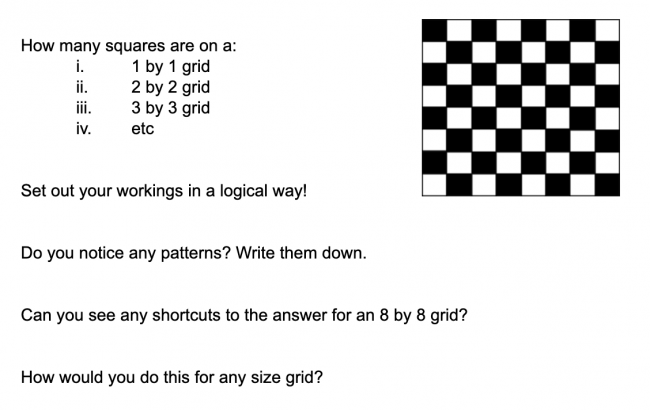# Squares on a Chessboard

Preview:Students investigate how many squares there are on a chessboard of any size.

Project
Big Idea:
Grade 8: Number represents, describes, and compares the quantities of ratios, rates, and percents.
Grade 9: The principles and processes underlying operations with numbers apply equally to algebraic situations and can be described and analyzed.
Curricular Competency:
Reasoning and analyzing: Use reasoning to explore and make connections
Reasoning and analyzing: Model mathematics in contextualized experiences
Understanding and solving: Develop and use multiple strategies to engage in problem solving
Communicating and representing: Communicate mathematical thinking in many ways
Communicating and representing: Explain and justify mathematical ideas and decisions